GeeksforGeeks App
Open AppBrowser
Continue

# Union-Find Algorithm | (Union By Rank and Find by Optimized Path Compression)

Check whether a given graph contains a cycle or not.

Example:

`Input: `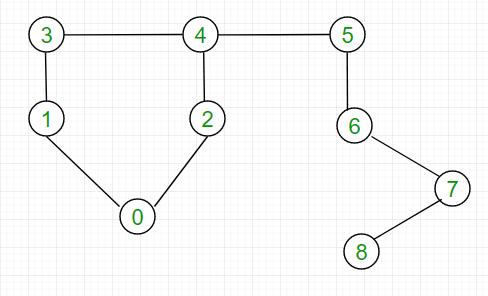```Output: Graph contains Cycle.

Input: ```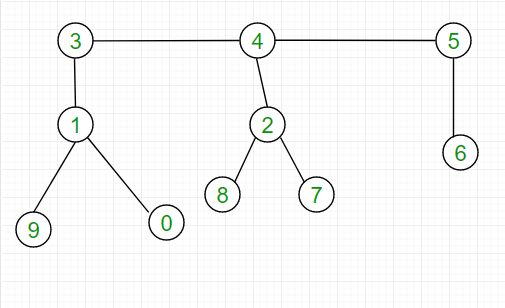`Output: Graph does not contain Cycle.`

Prerequisites: Disjoint Set (Or Union-Find), Union By Rank and Path Compression
We have already discussed union-find to detect cycle. Here we discuss find by path compression, where it is slightly modified to work faster than the original method as we are skipping one level each time we are going up the graph. Implementation of find function is iterative, so there is no overhead involved. Time complexity of optimized find function is O(log*(n)), i.e iterated logarithm, which converges to O(1) for repeated calls.
Proof of log*(n) complexity of Union-Find

Explanation of find function:

Take Example 1 to understand find function:
(1)call find(8) for first time and mappings will be done like this: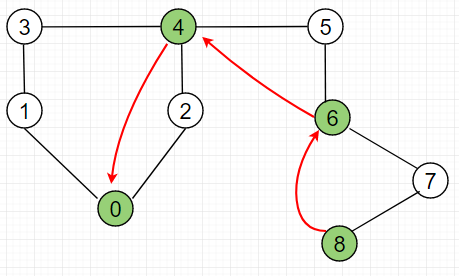It took 3 mappings for find function to get the root of node 8. Mappings are illustrated below:
From node 8, skipped node 7, Reached node 6.
From node 6, skipped node 5, Reached node 4.
From node 4, skipped node 2, Reached node 0.
(2)call find(8) for second time and mappings will be done like this: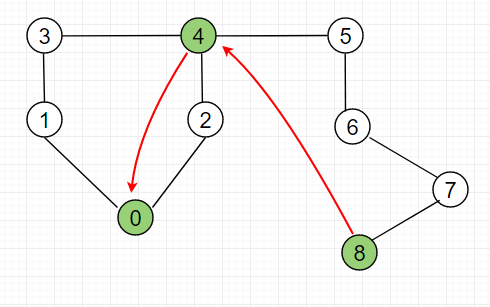It took 2 mappings to find function to get the root of node 8. Mappings are illustrated below:
From node 8, skipped node 5, node 6, and node 7, Reached node 4.
From node 4, skipped node 2, Reached node 0.
(3)call find(8) for third time and mappings will be done like this: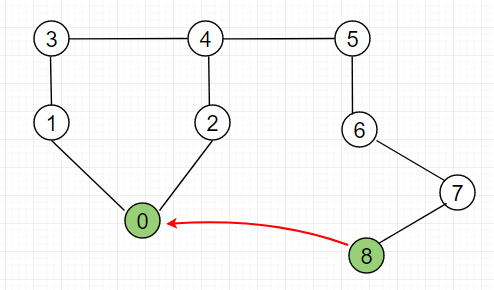Finally, we see it took only 1 mapping for find function to get the root of node 8. Mappings are illustrated below:
From node 8, skipped node 5, node 6, node 7, node 4, and node 2, Reached node 0.
That is how it converges path from certain mappings to single mapping.

Explanation of example 1:

```Initially array size and Arr look like:
Arr = {0, 1, 2, 3, 4, 5, 6, 7, 8}
size = {1, 1, 1, 1, 1, 1, 1, 1, 1}
Consider the edges in the graph, and add them one by one to the disjoint-union set as follows:
Edge 1: 0-1
find(0)=>0, find(1)=>1, both have different root parent
Put these in single connected component as currently they doesn't belong to different connected components.
Arr=0, size=2;
Edge 2: 0-2
find(0)=>0, find(2)=>2, both have different root parent
Arr=0, size=3;
Edge 3: 1-3
find(1)=>0, find(3)=>3, both have different root parent
Arr=0, size=3;
Edge 4: 3-4
find(3)=>1, find(4)=>4, both have different root parent
Arr=0, size=4;
Edge 5: 2-4
find(2)=>0, find(4)=>0, both have same root parent
Hence, There is a cycle in graph.
We stop further checking for cycle in graph.
```

Implementation:

## C++

 `// CPP program to implement Union-Find with union``// by rank and path compression.``#include ``using` `namespace` `std;` `const` `int` `MAX_VERTEX = 101;` `// Arr to represent parent of index i``int` `Arr[MAX_VERTEX];` `// Size to represent the number of nodes``// in subgraph rooted at index i``int` `size[MAX_VERTEX];` `// set parent of every node to itself and``// size of node to one``void` `initialize(``int` `n)``{``    ``for` `(``int` `i = 0; i <= n; i++) {``        ``Arr[i] = i;``        ``size[i] = 1;``    ``}``}` `// Each time we follow a path, find function``// compresses it further until the path length``// is greater than or equal to 1.``int` `find(``int` `i)``{``    ``// while we reach a node whose parent is``    ``// equal to itself``    ``while` `(Arr[i] != i)``    ``{``        ``Arr[i] = Arr[Arr[i]]; ``// Skip one level``        ``i = Arr[i]; ``// Move to the new level``    ``}``    ``return` `i;``}` `// A function that does union of two nodes x and y``// where xr is root node  of x and yr is root node of y``void` `_union(``int` `xr, ``int` `yr)``{``    ``if` `(size[xr] < size[yr]) ``// Make yr parent of xr``    ``{``        ``Arr[xr] = Arr[yr];``        ``size[yr] += size[xr];``    ``}``    ``else` `// Make xr parent of yr``    ``{``        ``Arr[yr] = Arr[xr];``        ``size[xr] += size[yr];``    ``}``}` `// The main function to check whether a given``// graph contains cycle or not``int` `isCycle(vector<``int``> adj[], ``int` `V)``{``    ``// Iterate through all edges of graph, find``    ``// nodes connecting them.``    ``// If root nodes of both are same, then there is``    ``// cycle in graph.``    ``for` `(``int` `i = 0; i < V; i++) {``        ``for` `(``int` `j = 0; j < adj[i].size(); j++) {``            ``int` `x = find(i); ``// find root of i``            ``int` `y = find(adj[i][j]); ``// find root of adj[i][j]` `            ``if` `(x == y)``                ``return` `1; ``// If same parent``            ``_union(x, y); ``// Make them connect``        ``}``    ``}``    ``return` `0;``}` `// Driver program to test above functions``int` `main()``{``    ``int` `V = 3;` `    ``// Initialize the values for array Arr and Size``    ``initialize(V);` `    ``/* Let us create following graph``         ``0``        ``|  \``        ``|    \``        ``1-----2 */` `    ``vector<``int``> adj[V]; ``// Adjacency list for graph` `    ``adj.push_back(1);``    ``adj.push_back(2);``    ``adj.push_back(2);` `    ``// call is_cycle to check if it contains cycle``    ``if` `(isCycle(adj, V))``        ``cout << ``"Graph contains Cycle.\n"``;``    ``else``        ``cout << ``"Graph does not contain Cycle.\n"``;` `    ``return` `0;``}`

## Java

 `// Java program to implement Union-Find with union``// by rank and path compression``import` `java.util.*;` `class` `GFG``{``static` `int` `MAX_VERTEX = ``101``;` `// Arr to represent parent of index i``static` `int` `[]Arr = ``new` `int``[MAX_VERTEX];` `// Size to represent the number of nodes``// in subgraph rooted at index i``static` `int` `[]size = ``new` `int``[MAX_VERTEX];` `// set parent of every node to itself and``// size of node to one``static` `void` `initialize(``int` `n)``{``    ``for` `(``int` `i = ``0``; i <= n; i++)``    ``{``        ``Arr[i] = i;``        ``size[i] = ``1``;``    ``}``}` `// Each time we follow a path, find function``// compresses it further until the path length``// is greater than or equal to 1.``static` `int` `find(``int` `i)``{``    ``// while we reach a node whose parent is``    ``// equal to itself``    ``while` `(Arr[i] != i)``    ``{``        ``Arr[i] = Arr[Arr[i]]; ``// Skip one level``        ``i = Arr[i]; ``// Move to the new level``    ``}``    ``return` `i;``}` `// A function that does union of two nodes x and y``// where xr is root node of x and yr is root node of y``static` `void` `_union(``int` `xr, ``int` `yr)``{``    ``if` `(size[xr] < size[yr]) ``// Make yr parent of xr``    ``{``        ``Arr[xr] = Arr[yr];``        ``size[yr] += size[xr];``    ``}``    ``else` `// Make xr parent of yr``    ``{``        ``Arr[yr] = Arr[xr];``        ``size[xr] += size[yr];``    ``}``}` `// The main function to check whether a given``// graph contains cycle or not``static` `int` `isCycle(Vector adj[], ``int` `V)``{``    ``// Iterate through all edges of graph,``    ``// find nodes connecting them.``    ``// If root nodes of both are same,``    ``// then there is cycle in graph.``    ``for` `(``int` `i = ``0``; i < V; i++)``    ``{``        ``for` `(``int` `j = ``0``; j < adj[i].size(); j++)``        ``{``            ``int` `x = find(i); ``// find root of i``            ` `            ``// find root of adj[i][j]``            ``int` `y = find(adj[i].get(j));` `            ``if` `(x == y)``                ``return` `1``; ``// If same parent``            ``_union(x, y); ``// Make them connect``        ``}``    ``}``    ``return` `0``;``}` `// Driver Code``public` `static` `void` `main(String[] args)``{``    ``int` `V = ``3``;` `    ``// Initialize the values for array Arr and Size``    ``initialize(V);` `    ``/* Let us create following graph``        ``0``        ``| \``        ``| \``        ``1-----2 */` `    ``// Adjacency list for graph``    ``Vector []adj = ``new` `Vector[V];``    ``for``(``int` `i = ``0``; i < V; i++)``        ``adj[i] = ``new` `Vector();` `    ``adj[``0``].add(``1``);``    ``adj[``0``].add(``2``);``    ``adj[``1``].add(``2``);` `    ``// call is_cycle to check if it contains cycle``    ``if` `(isCycle(adj, V) == ``1``)``        ``System.out.print(``"Graph contains Cycle.\n"``);``    ``else``        ``System.out.print(``"Graph does not contain Cycle.\n"``);``    ``}``}` `// This code is contributed by PrinciRaj1992`

## Python3

 `# Python3 program to implement Union-Find``# with union by rank and path compression.` `# set parent of every node to itself``# and size of node to one``def` `initialize(n):``    ``global` `Arr, size``    ``for` `i ``in` `range``(n ``+` `1``):``        ``Arr[i] ``=` `i``        ``size[i] ``=` `1` `# Each time we follow a path, find``# function compresses it further``# until the path length is greater``# than or equal to 1.``def` `find(i):``    ``global` `Arr, size``    ` `    ``# while we reach a node whose``    ``# parent is equal to itself``    ``while` `(Arr[i] !``=` `i):``        ``Arr[i] ``=` `Arr[Arr[i]] ``# Skip one level``        ``i ``=` `Arr[i] ``# Move to the new level``    ``return` `i` `# A function that does union of two``# nodes x and y where xr is root node``# of x and yr is root node of y``def` `_union(xr, yr):``    ``global` `Arr, size``    ``if` `(size[xr] < size[yr]): ``# Make yr parent of xr``        ``Arr[xr] ``=` `Arr[yr]``        ``size[yr] ``+``=` `size[xr]``    ``else``: ``# Make xr parent of yr``        ``Arr[yr] ``=` `Arr[xr]``        ``size[xr] ``+``=` `size[yr]` `# The main function to check whether``# a given graph contains cycle or not``def` `isCycle(adj, V):``    ``global` `Arr, size``    ` `    ``# Iterate through all edges of graph,``    ``# find nodes connecting them.``    ``# If root nodes of both are same,``    ``# then there is cycle in graph.``    ``for` `i ``in` `range``(V):``        ``for` `j ``in` `range``(``len``(adj[i])):``            ``x ``=` `find(i) ``# find root of i``            ``y ``=` `find(adj[i][j]) ``# find root of adj[i][j]` `            ``if` `(x ``=``=` `y):``                ``return` `1` `# If same parent``            ``_union(x, y) ``# Make them connect``    ``return` `0` `# Driver Code``MAX_VERTEX ``=` `101` `# Arr to represent parent of index i``Arr ``=` `[``None``] ``*` `MAX_VERTEX` `# Size to represent the number of nodes``# in subgraph rooted at index i``size ``=` `[``None``] ``*` `MAX_VERTEX` `V ``=` `3` `# Initialize the values for array``# Arr and Size``initialize(V)` `# Let us create following graph``#     0``# | \``# | \``# 1-----2` `# Adjacency list for graph``adj ``=` `[[] ``for` `i ``in` `range``(V)]` `adj[``0``].append(``1``)``adj[``0``].append(``2``)``adj[``1``].append(``2``)` `# call is_cycle to check if it``# contains cycle``if` `(isCycle(adj, V)):``    ``print``(``"Graph contains Cycle."``)``else``:``    ``print``(``"Graph does not contain Cycle."``)` `# This code is contributed by PranchalK`

## C#

 `// C# program to implement Union-Find``// with union by rank and path compression``using` `System;``using` `System.Collections.Generic;``    ` `class` `GFG``{``static` `int` `MAX_VERTEX = 101;` `// Arr to represent parent of index i``static` `int` `[]Arr = ``new` `int``[MAX_VERTEX];` `// Size to represent the number of nodes``// in subgraph rooted at index i``static` `int` `[]size = ``new` `int``[MAX_VERTEX];` `// set parent of every node to itself``// and size of node to one``static` `void` `initialize(``int` `n)``{``    ``for` `(``int` `i = 0; i <= n; i++)``    ``{``        ``Arr[i] = i;``        ``size[i] = 1;``    ``}``}` `// Each time we follow a path,``// find function compresses it further``// until the path length is greater than``// or equal to 1.``static` `int` `find(``int` `i)``{``    ``// while we reach a node whose``    ``// parent is equal to itself``    ``while` `(Arr[i] != i)``    ``{``        ``Arr[i] = Arr[Arr[i]]; ``// Skip one level``        ``i = Arr[i]; ``// Move to the new level``    ``}``    ``return` `i;``}` `// A function that does union of``// two nodes x and y where xr is``// root node of x and yr is root node of y``static` `void` `_union(``int` `xr, ``int` `yr)``{``    ``if` `(size[xr] < size[yr]) ``// Make yr parent of xr``    ``{``        ``Arr[xr] = Arr[yr];``        ``size[yr] += size[xr];``    ``}``    ``else` `// Make xr parent of yr``    ``{``        ``Arr[yr] = Arr[xr];``        ``size[xr] += size[yr];``    ``}``}` `// The main function to check whether``// a given graph contains cycle or not``static` `int` `isCycle(List<``int``> []adj, ``int` `V)``{``    ``// Iterate through all edges of graph,``    ``// find nodes connecting them.``    ``// If root nodes of both are same,``    ``// then there is cycle in graph.``    ``for` `(``int` `i = 0; i < V; i++)``    ``{``        ``for` `(``int` `j = 0; j < adj[i].Count; j++)``        ``{``            ``int` `x = find(i); ``// find root of i``            ` `            ``// find root of adj[i][j]``            ``int` `y = find(adj[i][j]);` `            ``if` `(x == y)``                ``return` `1; ``// If same parent``            ``_union(x, y); ``// Make them connect``        ``}``    ``}``    ``return` `0;``}` `// Driver Code``public` `static` `void` `Main(String[] args)``{``    ``int` `V = 3;` `    ``// Initialize the values for``    ``// array Arr and Size``    ``initialize(V);` `    ``/* Let us create following graph``        ``0``        ``| \``        ``| \``        ``1-----2 */` `    ``// Adjacency list for graph``    ``List<``int``> []adj = ``new` `List<``int``>[V];``    ``for``(``int` `i = 0; i < V; i++)``        ``adj[i] = ``new` `List<``int``>();` `    ``adj.Add(1);``    ``adj.Add(2);``    ``adj.Add(2);` `    ``// call is_cycle to check if it contains cycle``    ``if` `(isCycle(adj, V) == 1)``        ``Console.Write(``"Graph contains Cycle.\n"``);``    ``else``        ``Console.Write(``"Graph does not contain Cycle.\n"``);``    ``}``}` `// This code is contributed by Rajput-Ji`

## Javascript

 ``

Output

`Graph contains Cycle.`

Complexity Analysis:

• Time Complexity(Find) : O(log*(n))
• Time Complexity(union) : O(1)
• Auxiliary Space: O(Max_Vertex)

My Personal Notes arrow_drop_up